StatLect

# Discrete random vector

A discrete random vector is a random vector that can take either a finite or an infinite but countable number of values.## Definition

The following is a possible definition.

Definition A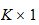random vectoris said to be discrete if and only if the set of values it can take has either a finite or an infinite but countable number of elements, and there exists a function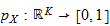, called joint probability mass function, such that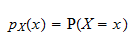where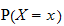is the probability thattakes the value.

A random vector that is discrete is also said to possess a multivariate discrete distribution.

## Example

Suppose a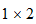random vectorcan take only one of three values,,ordefined by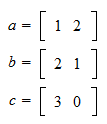and thathas probabilityof being observed, whileandhave probability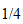. Then,is discrete because it can take only finitely many values. Its joint probability mass function is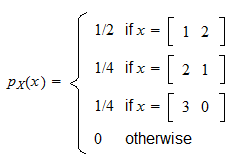When the two entries ofare denoted byand, the joint probability mass function can also be written as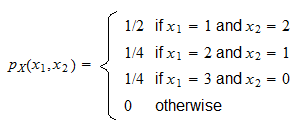## More details

The lecture entitled Random vectors provides a more complete treatment of discrete random vectors and joint probability mass functions.

Previous entry: Discrete random variable

Next entry: Distribution function